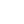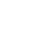• Index

• Products+

• Solution+

• Cases+

• AI Lab+

• Investor+

• ResourceCenter+

• Contact+

• Trial+

### A Credit Score Model Based on XGBoost (Part 2)

So far, we have introduced the theory of xbboost. Now we will use this algorithm to train the data in this paper. In the process of training, we need to adjust the parameters to get the optimal parameters.

This paper uses boost package to train the model, and combines the grid search function of sklearn package to find the optimal parameters. When adjusting parameters, a fixed value is given for each parameter, and then the range of values of parameters to be adjusted is given, while other parameter values are kept unchanged. Therefore, the AUC score of the model is calculated under different values of the parameter. The parameter value corresponding to the maximum AUC score is the best value of the parameter, The optimal parameter value in this time replaces the given value of the parameter in the model and then adjusts the next parameter according to this method.